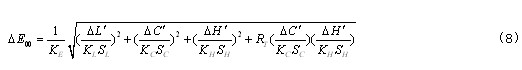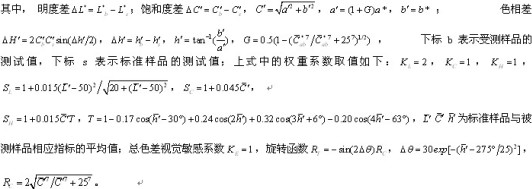# Research on Small Color Difference Detection of Transparent Magenta Plastic Based on CIEDE2000

Chromatic aberration is an important test indicator for plastic products. The detection of chromatic aberration of traditional plastic products is based on the calculation of the chromatic aberration between different plastic products by measuring the transmittance or reflectance of materials at three specific wavelengths. This method has certain limitations . The current research is more CIELAB color space, it is a uniform color space, but not ideal, CIELAB color difference formula calculation results and visual perception can not always be consistent; and CIEDE2000 color difference formula is considered to be the best theoretical color difference The formula, which is defined in the modified CIELAB space, not only has a better brightness, chroma, and hue weighting function for evaluating small chromatic aberrations, but also has correction blue and green difference latitude parameters. With the deepening of theoretical research [2-5], the application field of CIEDE2000 color difference formula has been continuously expanded, and there are related researches in the fields of industry, agriculture, medical treatment and military [6-11].

In this paper, according to the CIE (International Commission on Illumination) recommended equally spaced wavelength method, the transmittance of the sample is measured every 5 nm in the visible wavelength range of 380-800 nm, and the tristimulus value is obtained by using the D65 illuminator. Then calculate their color difference according to CIE1976LAB and CIEDE2000 respectively. And the results of the respective calculations are compared with the visual effects of the human eye, and the color difference evaluation method conforming to the transparent magenta plastic is selected from the comparison results.

1 Basic principles

Spectrophotometers do not measure color directly, but rather compare the reference of certain known spectral properties, such as (spreading it uniformly on the inner wall of the integrating sphere as an approximately complete diffuse reflector) and transmitting the sample at the same wavelength. Radiated power, which in turn measures the transmittance of the sample at a selected wavelength (spectral radiance factor). The tristimulus values â€‹â€‹of the samples were calculated according to the CIE (International Commission on Illumination) chroma system tristimulus values, and then substituted into the relevant color difference formula to compare the color difference between the two samples.

The CIE Chromaticity System tristimulus value can be obtained by multiplying the color stimulation function by the CIE spectral tristimulus value and integrating over the entire visible spectrum: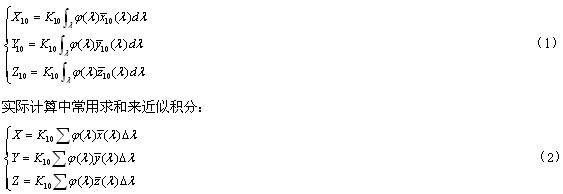In equation (1)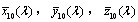It is the CIE 1964 supplementary standard chroma observer spectral tristimulus value specified by CIE (obtained by the table). The constant is a normalization coefficient, which is obtained when the self-luminous object is adjusted to a light source Y value (where Y is the light reflectance of the object) to 100. For a non-self-illuminating object, the Y value of the selected standard illuminant is adjusted to 100, that is, the Y value of the fully diffuse reflector and the ideal transmissive object is adjusted to 100, which can be obtained by the following formula: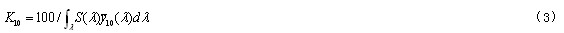The choice in the formula varies according to the spectral characteristics of the measured object and the calculation accuracy. When taken, accurate results can be given in some cases; when taken, accurate results can be given in most cases.

It is called the color stimulating function, that is, the light energy that enters the human eye to produce a color sensation. When the measured object is a non-self-illuminating body, the color stimulating functions of the transparent or opaque body are: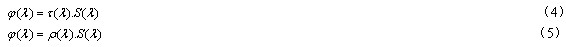Where is the spectral transmittance of the object, the spectral reflectance of the object, and the relative spectral power of the illumination source. Since the product is used in an outdoor environment, the spectral data is used in the following calculations using the D65 illuminator.

The chrominance values â€‹â€‹in the color mixing system can be expressed as Y, x, y.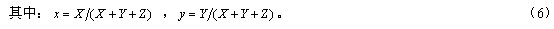2 Calculation formula and test data

2.1 CIE 1976 LAB color difference formula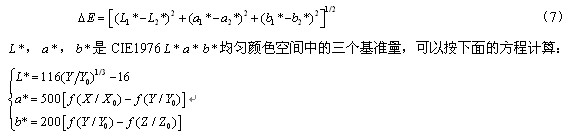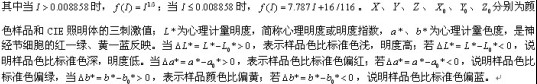2.2 CIE DE 2000 color difference formula